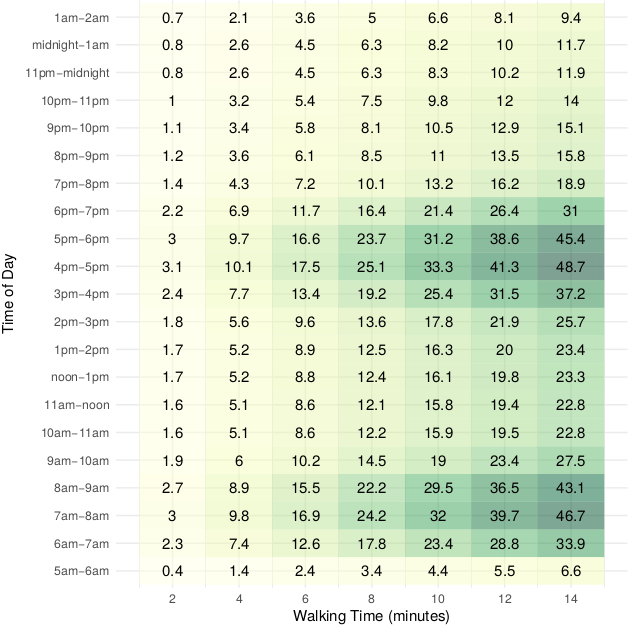# Comparing Neighbourhood-Level Transit Availability:

How does it vary for different walking times and by time of day for Winnipeg?Each cell in the figure above shows $$\Omega_{i,\tau,\theta}$$, the average number of unique transit trips someone living in a zone, $$i$$, can reach within a $$\theta$$ minute walk between the times of $$\tau_a$$ and $$\tau_b$$ (e.g. $$\tau_a$$ = 7am and $$\tau_b$$ = 9am for analyzing transit availability during a morning peak period).

$$\Omega_{i,\tau,\theta}$$ is calculated as follows:

1 - For every zone $$i$$, find all transit stops, $$j$$, which have a $$\theta$$ minute network walking buffer, $$A_{j,\theta}$$, which intersects the zone, $$A_i$$. $J = \{\hspace{1mm}j : A_{j,\theta} \cap A_i > 0\hspace{1mm}\}$

2 - Select all trips, $$t$$, which service these stops within the time period of analysis. If a trip, $$t$$, serves more than one intersecting transit stop, $$j$$, it is only counted once. $T = \{\hspace{1mm}t : \tau_{a} \leq \tau_{t,j} \leq \tau_{b}\hspace{1mm} \}$

3 - For each trip, $$t$$, select area of the trip which serves the zone, $$A_{i,t,\theta}$$, which is the combined area of the $$\theta$$ minute walking network buffer for each of the stops that the trip serves, without doubly counting any overlap between network buffers. $A_{i,t,\theta} = \bigcup \hspace{0mm} A_{j,\theta} \hspace{2mm} \forall \{j : \tau_{a} \leq \tau_{t,j} \leq \tau_{b}\}$

4 - For each trip, $$t$$, compute the ratio of the area served by the trip that intersects the zone, $$A_{i,t,\theta} \cap A_i$$, by the total area of the zone, $$A_i$$. i.e. this is the proportion of the zone, $$i$$, that can reach trip, $$t$$, for a specific walking threshold, $$\theta$$. Then sum the ratios to find the average number of trips reachable in each zone for the time period, $$\tau$$. $\Omega_{i,\tau,\theta} = k \sum_{t \in T}^{} \frac{(A_{i,t,\theta} \cap A_i)}{A_i}$ $$k$$ is an optional conversion factor. i.e. if $$\tau_a$$ = 4:00pm and $$\tau_b$$ = 8:00pm then $$k = 0.25$$ to present the result as per hour metric.

Since $$\Omega_{i,\tau,\theta}$$ is linked to spatial units, it can easily be mapped to compare between different inputs of $$\tau$$ and $$\theta$$.

Region wide population averages can be computed as follows, where $$p_i$$ is the population in each zone, $$i$$. This can be computed for the overall population, or sub-groups in order to examine how transit availability differs by demographics of socio-economic status.

$\bar\Omega_{\tau,\theta,p} = \frac{\sum_i p_{i} \Omega_{i,\tau,\theta}}{\sum_i p_i}$

Data can also be summarized as Gini coefficients in order to analyze spatial inequalities of transit availability. The Gini has been used in other studies to examine how transit availability is distributed among the overall population. The Gini is computed as follows.

$G_\Omega = \frac{\sum_{m=1}^{M} \sum_{N=1}^{N} | \Omega_m - \Omega_n |}{2 N \sum_{m=1}^{M} \Omega_m}$

Here’s differences in the Gini